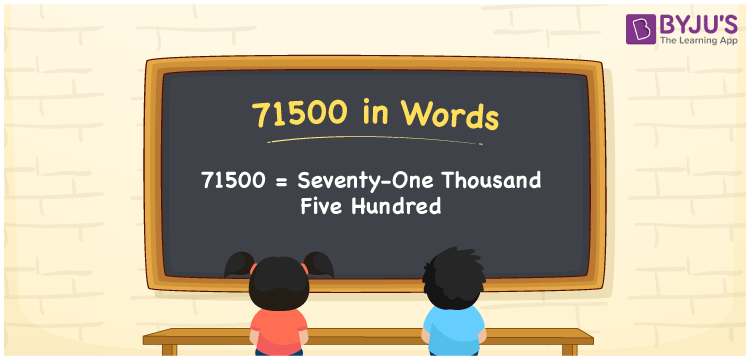# 71500 in Words

71500 in words is written as Seventy-one thousand five hundred. In both the International System of Numerals and the Indian System of Numerals, 71500 is written as Seventy-one thousand five hundred. The number 71500 is a Cardinal Number as it represents some quantity. For example, “that laptop costs 71500 rupees”.

 71500 in Words Seventy-one thousand five hundred Seventy-one thousand five hundred in Number 71500

## 71500 in English Words

71500 in English words is read as “Seventy-one thousand five hundred.”## How to Write 71500 in Words?

To write 71500 in words, we shall use the place value chart. In the place value chart, put 7 in the ten thousands, 1 in the thousands, 5 in the hundreds and put 0 in the rest. Let us make a place value chart to write the number 71500 in words.

 Ten Thousands Thousands Hundreds Tens Ones 7 1 5 0 0

Thus, we can write the expanded form as

7 × Ten Thousand + 1 × Thousand + 5 × Hundred + 0 × Ten + 0 × One

= 7 × 10000 + 1 × 1000 + 5 × 100 + 0 × 10 + 0 × 1

= 70000 + 1000 + 500 + 0 + 0

= 71500

= Seventy-one thousand five hundred.

71500 is a natural number, the successor of 71499 and the predecessor of 71501.

71500 in words – Seventy-one thousand five hundred

• Is 71500 an odd number? – No
• Is 71500 an even number? – Yes
• Is 71500 a perfect square number? – No
• Is 71500 a perfect cube number? – No
• Is 71500 a prime number? – No
• Is 71500 a composite number? – Yes

## Frequently Asked Questions on 71500 in Words

Q1

### How to write 71500 in words?

71500 in words is written as Seventy-one thousand five hundred.
Q2

### How to write 71500 in the International and Indian System of Numerals?

In both, the system of numerals, 71500 in words, is written as Seventy-one thousand five hundred.
Q3

### How to write 71500 in a place value chart?

In the place value chart, write 7 in the ten thousands, 1 in the thousands, 5 in the hundreds and 0 in the tens and ones, respectively.